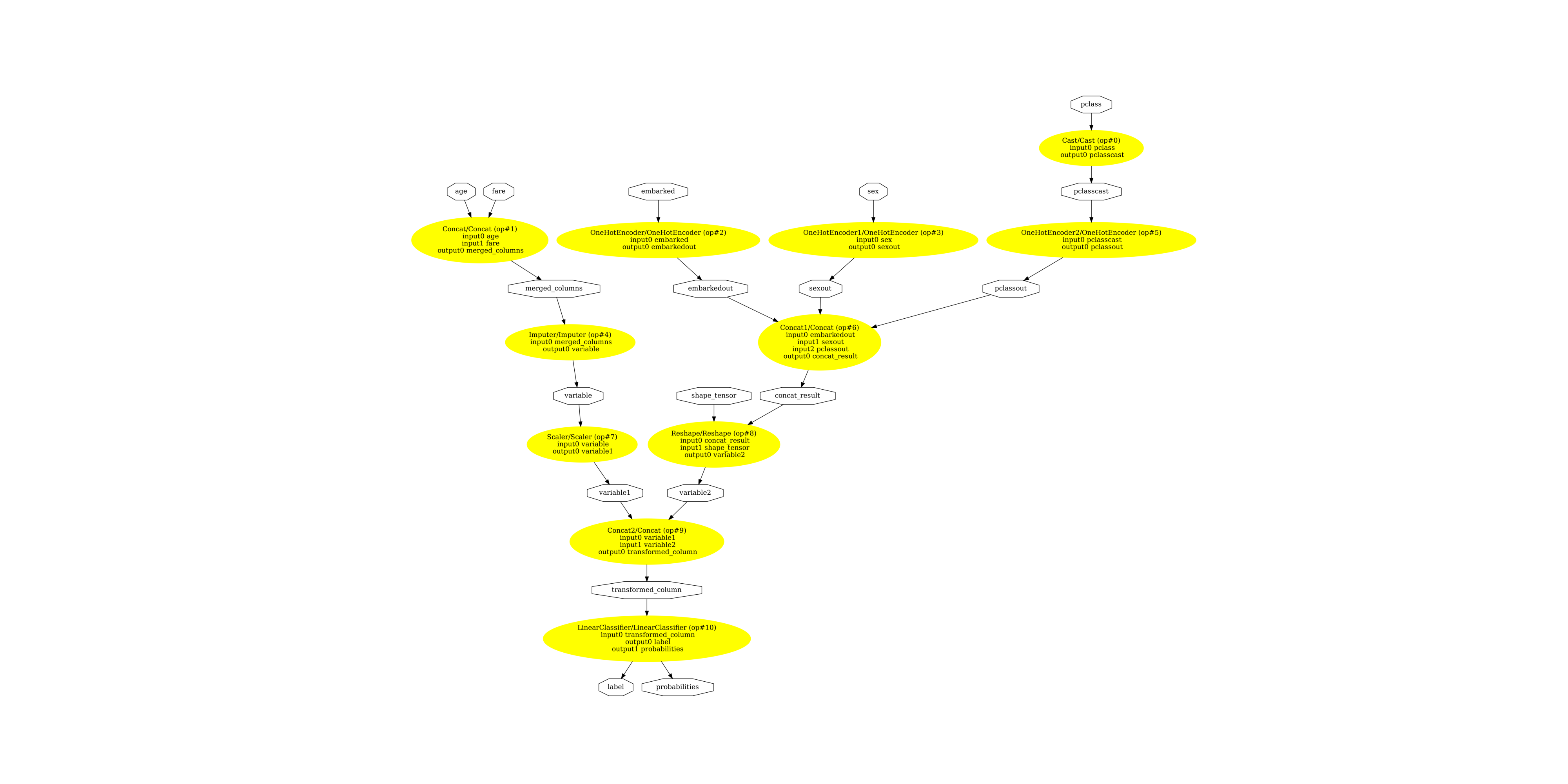# Convert a pipeline with ColumnTransformer#

scikit-learn recently shipped ColumnTransformer which lets the user define complex pipeline where each column may be preprocessed with a different transformer. sklearn-onnx still works in this case as shown in Section Convert complex pipelines.

## Create and train a complex pipeline#

We reuse the pipeline implemented in example Column Transformer with Mixed Types. There is one change because ONNX-ML Imputer does not handle string type. This cannot be part of the final ONNX pipeline and must be removed. Look for comment starting with `---` below.

```import os
import pprint
import pandas as pd
import numpy as np
from numpy.testing import assert_almost_equal
import onnx
from onnx.tools.net_drawer import GetPydotGraph, GetOpNodeProducer
import onnxruntime as rt
import matplotlib.pyplot as plt
import sklearn
from sklearn.compose import ColumnTransformer
from sklearn.pipeline import Pipeline
from sklearn.impute import SimpleImputer
from sklearn.preprocessing import StandardScaler, OneHotEncoder
from sklearn.linear_model import LogisticRegression
from sklearn.model_selection import train_test_split
import skl2onnx
from skl2onnx import convert_sklearn
from skl2onnx.common.data_types import FloatTensorType, StringTensorType
from skl2onnx.common.data_types import Int64TensorType

titanic_url = (
"https://raw.githubusercontent.com/amueller/"
"scipy-2017-sklearn/091d371/notebooks/datasets/titanic3.csv"
)
X = data.drop("survived", axis=1)
y = data["survived"]
print(data.dtypes)

# SimpleImputer on string is not available for
# string in ONNX-ML specifications.
# So we do it beforehand.
for cat in ["embarked", "sex", "pclass"]:
X[cat].fillna("missing", inplace=True)

X_train, X_test, y_train, y_test = train_test_split(X, y, test_size=0.2)

numeric_features = ["age", "fare"]
numeric_transformer = Pipeline(
steps=[("imputer", SimpleImputer(strategy="median")), ("scaler", StandardScaler())]
)

categorical_features = ["embarked", "sex", "pclass"]
categorical_transformer = Pipeline(
steps=[
# --- SimpleImputer is not available for strings in ONNX-ML specifications.
# ('imputer', SimpleImputer(strategy='constant', fill_value='missing')),
("onehot", OneHotEncoder(handle_unknown="ignore"))
]
)

preprocessor = ColumnTransformer(
transformers=[
("num", numeric_transformer, numeric_features),
("cat", categorical_transformer, categorical_features),
]
)

clf = Pipeline(
steps=[
("preprocessor", preprocessor),
("classifier", LogisticRegression(solver="lbfgs")),
]
)

clf.fit(X_train, y_train)
```
```pclass         int64
survived       int64
name          object
sex           object
age          float64
sibsp          int64
parch          int64
ticket        object
fare         float64
cabin         object
embarked      object
boat          object
body         float64
home.dest     object
dtype: object
```
```Pipeline(steps=[('preprocessor',
ColumnTransformer(transformers=[('num',
Pipeline(steps=[('imputer',
SimpleImputer(strategy='median')),
('scaler',
StandardScaler())]),
['age', 'fare']),
('cat',
Pipeline(steps=[('onehot',
OneHotEncoder(handle_unknown='ignore'))]),
['embarked', 'sex',
'pclass'])])),
('classifier', LogisticRegression())])```
In a Jupyter environment, please rerun this cell to show the HTML representation or trust the notebook.

## Define the inputs of the ONNX graph#

sklearn-onnx does not know the features used to train the model but it needs to know which feature has which name. We simply reuse the dataframe column definition.

```print(X_train.dtypes)
```
```pclass         int64
name          object
sex           object
age          float64
sibsp          int64
parch          int64
ticket        object
fare         float64
cabin         object
embarked      object
boat          object
body         float64
home.dest     object
dtype: object
```

After conversion.

```def convert_dataframe_schema(df, drop=None):
inputs = []
for k, v in zip(df.columns, df.dtypes):
if drop is not None and k in drop:
continue
if v == "int64":
t = Int64TensorType([None, 1])
elif v == "float64":
t = FloatTensorType([None, 1])
else:
t = StringTensorType([None, 1])
inputs.append((k, t))
return inputs

initial_inputs = convert_dataframe_schema(X_train)

pprint.pprint(initial_inputs)
```
```[('pclass', Int64TensorType(shape=[None, 1])),
('name', StringTensorType(shape=[None, 1])),
('sex', StringTensorType(shape=[None, 1])),
('age', FloatTensorType(shape=[None, 1])),
('sibsp', Int64TensorType(shape=[None, 1])),
('parch', Int64TensorType(shape=[None, 1])),
('ticket', StringTensorType(shape=[None, 1])),
('fare', FloatTensorType(shape=[None, 1])),
('cabin', StringTensorType(shape=[None, 1])),
('embarked', StringTensorType(shape=[None, 1])),
('boat', StringTensorType(shape=[None, 1])),
('body', FloatTensorType(shape=[None, 1])),
('home.dest', StringTensorType(shape=[None, 1]))]
```

Merging single column into vectors is not the most efficient way to compute the prediction. It could be done before converting the pipeline into a graph.

## Convert the pipeline into ONNX#

```try:
model_onnx = convert_sklearn(
clf, "pipeline_titanic", initial_inputs, target_opset=12
)
except Exception as e:
print(e)
```

Predictions are more efficient if the graph is small. That’s why the converter checks that there is no unused input. They need to be removed from the graph inputs.

```to_drop = {"parch", "sibsp", "cabin", "ticket", "name", "body", "home.dest", "boat"}
initial_inputs = convert_dataframe_schema(X_train, to_drop)
try:
model_onnx = convert_sklearn(
clf, "pipeline_titanic", initial_inputs, target_opset=12
)
except Exception as e:
print(e)
```

scikit-learn does implicit conversions when it can. sklearn-onnx does not. The ONNX version of OneHotEncoder must be applied on columns of the same type.

```initial_inputs = convert_dataframe_schema(X_train, to_drop)

model_onnx = convert_sklearn(clf, "pipeline_titanic", initial_inputs, target_opset=12)

# And save.
with open("pipeline_titanic.onnx", "wb") as f:
f.write(model_onnx.SerializeToString())
```

## Compare the predictions#

Final step, we need to ensure the converted model produces the same predictions, labels and probabilities. Let’s start with scikit-learn.

```print("predict", clf.predict(X_test[:5]))
print("predict_proba", clf.predict_proba(X_test[:2]))
```
```predict [1 0 0 1 0]
predict_proba [[0.34887729 0.65112271]
[0.92762608 0.07237392]]
```

Predictions with onnxruntime. We need to remove the dropped columns and to change the double vectors into float vectors as onnxruntime does not support double floats. onnxruntime does not accept dataframe. inputs must be given as a list of dictionary. Last detail, every column was described not really as a vector but as a matrix of one column which explains the last line with the reshape.

```X_test2 = X_test.drop(to_drop, axis=1)
inputs = {c: X_test2[c].values for c in X_test2.columns}
for c in numeric_features:
inputs[c] = inputs[c].astype(np.float32)
for k in inputs:
inputs[k] = inputs[k].reshape((inputs[k].shape, 1))
```

We are ready to run onnxruntime.

```sess = rt.InferenceSession("pipeline_titanic.onnx", providers=["CPUExecutionProvider"])
pred_onx = sess.run(None, inputs)
print("predict", pred_onx[:5])
print("predict_proba", pred_onx[:2])
```
```predict [1 0 0 1 0]
predict_proba [{0: 0.34887731075286865, 1: 0.6511226892471313}, {0: 0.9276261329650879, 1: 0.0723738968372345}]
```

The output of onnxruntime is a list of dictionaries. Let’s swith to an array but that requires to convert again with an additional option zipmap.

```model_onnx = convert_sklearn(
clf,
"pipeline_titanic",
initial_inputs,
target_opset=12,
options={id(clf): {"zipmap": False}},
)
with open("pipeline_titanic_nozipmap.onnx", "wb") as f:
f.write(model_onnx.SerializeToString())

sess = rt.InferenceSession(
"pipeline_titanic_nozipmap.onnx", providers=["CPUExecutionProvider"]
)
pred_onx = sess.run(None, inputs)
print("predict", pred_onx[:5])
print("predict_proba", pred_onx[:2])
```
```predict [1 0 0 1 0]
predict_proba [[0.3488773  0.6511227 ]
[0.92762613 0.0723739 ]]
```

Let’s check they are the same.

```assert_almost_equal(clf.predict_proba(X_test), pred_onx)
```

## Display the ONNX graph#

Finally, let’s see the graph converted with sklearn-onnx.

```pydot_graph = GetPydotGraph(
model_onnx.graph,
name=model_onnx.graph.name,
rankdir="TB",
node_producer=GetOpNodeProducer(
"docstring", color="yellow", fillcolor="yellow", style="filled"
),
)
pydot_graph.write_dot("pipeline_titanic.dot")

os.system("dot -O -Gdpi=300 -Tpng pipeline_titanic.dot")

fig, ax = plt.subplots(figsize=(40, 20))
ax.imshow(image)
ax.axis("off")
``````(-0.5, 6901.5, 6049.5, -0.5)
```

Versions used for this example

```print("numpy:", np.__version__)
print("scikit-learn:", sklearn.__version__)
print("onnx: ", onnx.__version__)
print("onnxruntime: ", rt.__version__)
print("skl2onnx: ", skl2onnx.__version__)
```
```numpy: 1.23.5
scikit-learn: 1.4.dev0
onnx:  1.15.0
onnxruntime:  1.16.0+cu118
skl2onnx:  1.15.0
```

Total running time of the script: (0 minutes 8.095 seconds)

Gallery generated by Sphinx-Gallery